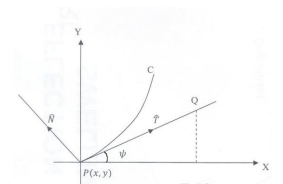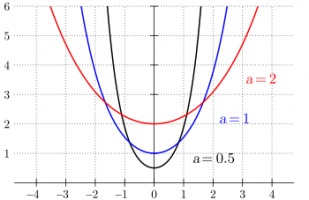# Velocity and acceleration

#### Dhamnekar Winod

##### Junior Member
A particle moves along a catenary $$\displaystyle s=c \tan{(\psi)}.$$The direction of acceleration at any point makes equal angles with the tangent and normal to the path at that point. If the speed at vertex $$\displaystyle (\psi = 0)$$ be $$\displaystyle 𝑣_0$$ , show that the magnitude of velocity and acceleration at any point are given by $$\displaystyle 𝑣_0 𝑒^{\psi}$$ and $$\displaystyle \frac{\sqrt{2}}{c} (𝑣_0)^2𝑒 ^{2\psi} \cos^2{(\psi)}$$ respectively.

#### Subhotosh Khan

##### Super Moderator
Staff member
A particle moves along a catenary $$\displaystyle s=c \tan{(\psi)}.$$The direction of acceleration at any point makes equal angles with the tangent and normal to the path at that point. If the speed at vertex $$\displaystyle (\psi = 0)$$ be $$\displaystyle 𝑣_0$$ , show that the magnitude of velocity and acceleration at any point are given by $$\displaystyle 𝑣_0 𝑒^{\psi}$$ and $$\displaystyle \frac{\sqrt{2}}{c} (𝑣_0)^2𝑒 ^{2\psi} \cos^2{(\psi)}$$ respectively.
Please check the given equation for catenary. In books that I have, it is a hyperbolic function.

If I were to solve this problem, I would:

Sketch catenary, with the particle at an arbitrary position

Determine the directions of velocity and acc celebration

And continue.

Last edited:
•topsquark

#### Dhamnekar Winod

##### Junior Member
Please check the given equation for catenary. In books that I have, it is a hyperbolic function.

If I were to solve this problem, I would:

Sketch catenary, with the particle at an arbitrary position

Determine the directions of velocity and acc celebration

And continue.

Catenary equation can be derived alternatively using the trigonometric function given in the question.

#### Subhotosh Khan

##### Super Moderator
Staff member
Catenary equation can be derived alternatively using the trigonometric function given in the question.
OK - so use it.

What is the direction of acceleration of the particle?

#### Dhamnekar Winod

##### Junior Member
OK - so use it.

What is the direction of acceleration of the particle?
Let $$\displaystyle \vec{r}$$ be the position vector of point P along the curve $$\displaystyle c\tan{(\psi)}$$. Then $$\displaystyle \frac{d\vec{r}}{d\psi}$$ is a unit vector along a tangent and

velocity v of the particle at any point of the curve is given by $$\displaystyle \vec{v} =\frac{d\vec{r}}{dt}= \frac{d\vec{r}}{d\psi}\frac{d\psi}{dt}=v \hat{T}$$ where $$\displaystyle v=\frac{d\psi}{dt} ,\hat{T}=\frac{d\vec{r}}{d\psi}$$,

$$\displaystyle \frac{d\vec{r}}{dt}=\frac{c}{\cos^2{(\psi)}}$$

If all the above work is correct, i shall proceed further.

Last edited:

#### Subhotosh Khan

##### Super Moderator
Staff member
Let $$\displaystyle \vec{r}$$ be the position vector of point P along the curve $$\displaystyle c\tan{(\psi)}$$. Then $$\displaystyle \frac{d\vec{r}}{d\psi}$$ is a unit vector along a tangent and

velocity v of the particle at any point of the curve is given by $$\displaystyle \vec{v} =\frac{d\vec{r}}{dt}= \frac{d\vec{r}}{d\psi}\frac{d\psi}{dt}=v \hat{T}$$ where $$\displaystyle v=\frac{d\psi}{dt} ,\hat{T}=\frac{d\vec{r}}{d\psi}$$,

$$\displaystyle \frac{d\vec{r}}{dt}=\frac{c}{\cos^2{(\psi)}}$$

If all the above work is correct, i shall proceed further.
Please post a sketch of the catenary - locating the particle and describing $$\displaystyle \psi ,$$ r and s

#### Dr.Peterson

##### Elite Member
I had never heard of this, but I found that the equation you give is the "Whewell equation" for the catenary, using [imath]\psi[/imath] for the angle of the tangent to the curve and s for the arc length. That is something that would have been worth explaining along with the problem, in order to provide context and define variables. An equation without such context is meaningless.

#### Dhamnekar Winod

##### Junior Member
Suppose the following figures depicts the catenary $$\displaystyle s= a\cdot \tan{(\psi)}$$ where s is arc legth of the curve C and a (in question it is c) is the distance from origin to the dip of the catenary.Let $$\displaystyle \vec{r}$$ be the position vector at point P. $$\displaystyle \vec{r}= x(s)\hat{i} + y(s)\hat{j} + z(s)\hat{k}$$

$$\displaystyle \hat{T}$$ is a unit tangent vector = $$\displaystyle \frac{d\vec{r}}{ds} = \frac{dx}{ds}\hat{i} +\frac{dy}{ds}\hat{j} + \frac{dz}{ds}\hat{k}=\frac{1}{a\cdot\tan^2{(\psi)}}\times\left[ \frac{dx}{d\psi}\hat{i} + \frac{dy}{d\psi}\hat{j} + \frac{dz}{d\psi}\hat{k} \right]$$

Velocity $$\displaystyle \vec{v}$$ of the particle at any point of the curve is given by $$\displaystyle \vec{v}=\frac{d\vec{r}}{d\psi}= \frac{d\vec{r}}{ds}\cdot\frac{ds}{d\psi}$$

$$\displaystyle \frac{ds}{d\psi}= a\cdot (1+\tan^2{(\psi)})$$ which is the velocity but velocity given in the question is $$\displaystyle V_0e^{\psi}$$

I know $$\displaystyle e^{\psi} = \cosh{(\psi)} + \sinh{(\psi)}$$

$$\displaystyle \vec{A}= \frac{dv}{d\psi}\hat{T} + \frac{v^2}{\rho}\frac{d\hat{T}}{d\psi}$$ since radius of curvature is $$\displaystyle \rho= \frac{ds}{d\psi}$$

$$\displaystyle \hat{N}$$ is a unit normal vector which is $$\displaystyle \frac{d\hat{T}}{d\psi}$$

Now the question states the angle between acceleration vector($$\displaystyle \vec{A}$$) and $$\displaystyle \hat{T}$$ is equal to the angle between $$\displaystyle \vec{A}$$ and $$\displaystyle \hat{N}$$

How to answer this question now?